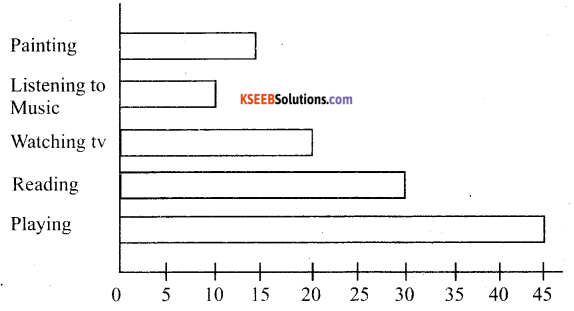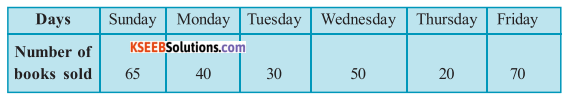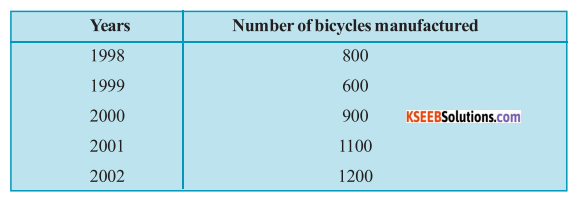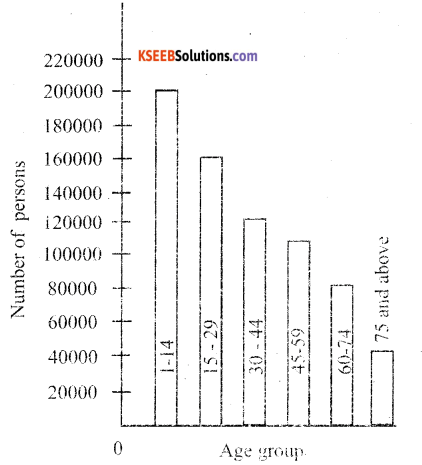# KSEEB Solutions for Class 6 Maths Chapter 9 Data Handling Ex 9.4

Students can Download Chapter 9 Data Handling Ex 9.4 Questions and Answers, Notes Pdf, KSEEB Solutions for Class 6 Maths helps you to revise the complete Karnataka State Board Syllabus and score more marks in your examinations.

## Karnataka State Syllabus Class 6 Maths Chapter 9 Data Handling Ex 9.4

Question 1.
A survey of 120 school students was done to find which activity they prefer to do in their free time.Draw a bar graph to illustrate the above data taking scale of 1 unit length = 5 students.
Which activity is preferred by most of the students other than playing?
Solution:
By taking a scale of 1 unit length = 5 students a bar graph of the above given data can be drawn as follows.The activity that is preferred by most of the students other than playing is reading story books.Question 2.
The number of Mathematics books sold by a shopkeeper on six consecutive days is shown below:Draw a bar graph to represent the above information choosing the scale of your choice.
Solution:
By taking a scale of 1 unit length = 10 books, a bar graph of the above given data can be drawn as followsQuestion 3.
Following table shows the number of bicycles manufactured in a factory during the years 1998 to 2002. Illustrate this data using a bar graph. Choose a scale of your choice.a) In which year was the maximum number of by cycles manufactured?
b) In which year was the minimum number of bicycles manufactured? Solution :
By taking a scale of 1 unit length = 100
Bicycles, a bar group of the above given data can be drawn as follows.a) The number of bicycles manufactured in 2002 was the maximum i.e. 1200
b) The number of bicycles manufactured in 1999 was the minimum .i.e. 600.Question 4.
Number of persons in various age groups in a town is given in the following table.Draw a bar graph to represent the above information and answer the following questions. (take 1 unit length = 20 thousands)
a) Which two age groups have same population?
b) All persons n the age group of 60 and above are called senior citizens. How many senior citizens are there in the town?
Solution :
By taking a scale of 1 unit length = 20 thousands, a bar group of the above given data can be drawn as follows.a) 30 – 44 and 45 are the two age groups which have the same population
b) It can be sinerred that senior citizens are the people who are either from age group 60 – 74 or from age group 45 and above.
Hence number of senior citizens = 80,000 + 40,000
= 1 lakhs 20 Thousand.

error: Content is protected !!